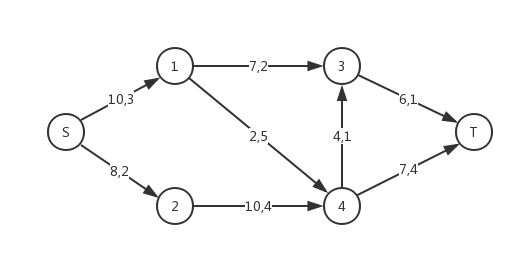# 最小费用最大流

## 定义## 做法

inline void add(int u,int v,int cap,int cost){
node[u].first = New(&node[u],&node[v],cap,cost);
node[v].first = New(&node[v],&node[u],0,-cost);
node[u].first->rev = node[v].first;
node[v].first->rev = node[u].first;
}
// 看不懂指针的朋友：这里就是反向边的消费是正向边的相反数的意思，


1. 网络初始流量为 $0$
2. 在当前的残量网络上求出从 $S$ 到 $T$ 的最短增广路，以每条弧的单位费用为边权。如果不存在则算法结束
3. 统计答案，更改流量，重复步骤 $2$

## 代码

#include
#include
#include
#include
#include
#include
#include
#include
#include
#include
#include
#include
#include

#define Re register
#define LL long long
#define U unsigned
#define FOR(i,a,b) for(Re int i = a;i <= b;++i)
#define ROF(i,a,b) for(Re int i = a;i >= b;--i)
#define SFOR(i,a,b,c) for(Re int i = a;i <= b;i+=c)
#define SROF(i,a,b,c) for(Re int i = a;i >= b;i-=c)
#define CLR(i,a) memset(i,a,sizeof(i))
#define BR printf("--------------------\n")
#define DEBUG(x) std::cerr << #x << '=' << x << std::endl

const int MAXN = 5000+5;
const int MAXM = 50000+5;

inline char nc(){
#define SIZE 100000
static char buf[SIZE],*p1 = buf+SIZE,*pend = buf+SIZE;
if(p1 == pend){
if(p1 == pend) return -1;
}
return *p1++;
}

x = 0;int flag = 1;
char ch = nc();
while(!isdigit(ch)){
if(ch == '-') flag = -1;
ch = nc();
}
while(isdigit(ch)){
x = (x<<1) + (x<<3) + (ch^'0');
ch = nc();
}
x *= flag;
}

struct Node{
struct Edge *first,*pre;
int dist,h;bool vis;
}node[MAXN];

struct Edge{
Node *s,*t;
Edge *next,*rev;
int flow,cap,cost;
}pool[(MAXM<<1)+3],*frog = pool;

Edge *New(Node *s,Node *t,int cap,int cost){
Edge *ret = ++frog;
*ret = (Edge){s,t,s->first,NULL,0,cap,cost};
return ret;
}

inline void add(int u,int v,int cap,int cost){
node[u].first = New(&node[u],&node[v],cap,cost);
node[v].first = New(&node[v],&node[u],0,-cost);
node[u].first->rev = node[v].first;
node[v].first->rev = node[u].first;
}

#define P std::pair
#define MP std::make_pair

int N,M,S,T;
int maxFlow,minCost;

bool spfa(Node *s,Node *t){
FOR(i,1,N) node[i].vis = false,node[i].dist = INT_MAX,node[i].pre = NULL;
s->vis = true;s->dist = 0;
std::queue q;q.push(s);
while(!q.empty()){
Node *v = q.front();q.pop();
v->vis = false;
for(Edge *e = v->first;e;e = e->next){
if(e->flow < e->cap){
if(e->t->dist > v->dist + e->cost){
e->t->dist = v->dist + e->cost;
e->t->pre = e;
if(!e->t->vis) q.push(e->t),e->t->vis = true;
}
}
}
}
return t->pre != NULL;
}

inline void work(){
while(spfa(&node[S],&node[T])){
int flow = INT_MAX;
for(Node *v = &node[T];v != &node[S];v = v->pre->s){
flow = std::min(flow,v->pre->cap-v->pre->flow);
}
maxFlow += flow;
for(Node *v = &node[T];v != &node[S];v = v->pre->s){
v->pre->flow += flow;
v->pre->rev->flow -= flow;
minCost += v->pre->cost * flow;
}
}
}

int main(){
//freopen("./LG/testdata.in","r",stdin);
// DEBUG(N);
while(M--){
int u,v,cap,cost;
}
work();
printf("%d %d\n",maxFlow,minCost);
return 0;
}


# 模型扩展

## 贴纸与玩偶### 代码

#include
#include
#include
#include
#include
#include
#include
#include
#include
#include
#include
#include
#include

#define Re register
#define LL long long
#define U unsigned
#define FOR(i,a,b) for(Re int i = a;i <= b;++i)
#define ROF(i,a,b) for(Re int i = a;i >= b;--i)
#define SFOR(i,a,b,c) for(Re int i = a;i <= b;i+=c)
#define SROF(i,a,b,c) for(Re int i = a;i >= b;i-=c)
#define CLR(i,a) memset(i,a,sizeof(i))
#define BR printf("--------------------\n")
#define DEBUG(x) std::cerr << #x << '=' << x << std::endl

const int MAXN = 1000+5;
const int MAXM = 10000+5;

struct Node{
struct Edge *first,*pre;
int dist;bool inq;
}node[MAXN];

struct Edge{
Node *s,*t;
Edge *next,*rev;
int cap,flow,cost;
}pool[MAXM<<1],*frog = pool;

Edge *New(Node *s,Node *t,int cap,int cost){
Edge *ret = ++frog;
*ret = (Edge){s,t,s->first,NULL,cap,0,cost};
return ret;
}

int N,M,S,T;

inline void add(int u,int v,int cap,int cost){
node[u].first = New(&node[u],&node[v],cap,cost);
node[v].first = New(&node[v],&node[u],0,-cost);
node[u].first->rev = node[v].first;
node[v].first->rev = node[u].first;
}

bool spfa(Node *s,Node *t){
FOR(i,0,N){
node[i].dist = INT_MAX;
node[i].inq = false;
node[i].pre = NULL;
}
std::queue q;
q.push(s);s->inq = true;s->dist = 0;
while(!q.empty()){
Node *v = q.front();q.pop();
v->inq = false;
for(Edge *e = v->first;e;e = e->next){
if(e->flow < e->cap && e->t->dist > v->dist + e->cost){
e->t->dist = v->dist + e->cost;
e->t->pre = e;
if(!e->t->inq){
q.push(e->t);e->t->inq = true;
}
}
}
}
return t->pre != NULL;
}

inline int mincost(){
int ret = 0;
while(spfa(&node[S],&node[T])){
int flow = INT_MAX;
for(Node *v = &node[T];v != &node[S];v = v->pre->s){
flow = std::min(flow,v->pre->cap-v->pre->flow);
}
for(Node *v = &node[T];v != &node[S];v = v->pre->s){
v->pre->flow += flow;
v->pre->rev->flow -= flow;
ret += v->pre->cost*flow;
}
}
return ret;
}

int a[MAXN],b[MAXN];
int h[MAXN][MAXM];
int n,m;

int work(int flag){
CLR(node,0);CLR(pool,0);frog = pool;
S = 0,T = n+m+1;
N = n+m+1;
FOR(i,1,n){
FOR(j,1,m){
}
}
return mincost()*flag;
}

int main(){
scanf("%d%d",&n,&m);
FOR(i,1,n) scanf("%d",a+i);
FOR(i,1,m) scanf("%d",b+i);
FOR(i,1,n) FOR(j,1,m) scanf("%d",&h[i][j]);
printf("%d\n",work(1));
printf("%d\n",work(-1));
return 0;
}


## 蒜头君的理发店### 代码

#include
#include
#include
#include
#include
#include
#include
#include
#include
#include
#include
#include
#include

#define Re register
#define LL long long
#define U unsigned
#define FOR(i,a,b) for(Re int i = a;i <= b;++i)
#define ROF(i,a,b) for(Re int i = a;i >= b;--i)
#define SFOR(i,a,b,c) for(Re int i = a;i <= b;i+=c)
#define SROF(i,a,b,c) for(Re int i = a;i >= b;i-=c)
#define CLR(i,a) memset(i,a,sizeof(i))
#define BR printf("--------------------\n")
#define DEBUG(x) std::cerr << #x << '=' << x << std::endl

const int MAXN = 1000+5;
const int MAXM = 1000000+5;

struct Node{
struct Edge *first,*pre;
int dist;bool inq;
}node[MAXN];

struct Edge{
Node *s,*t;
Edge *next,*rev;
int flow,cap,cost;
}pool[MAXM<<1],*frog = pool;

Edge *New(Node *s,Node *t,int cap,int cost){
Edge *ret = ++frog;
*ret = (Edge){s,t,s->first,NULL,0,cap,cost};
return ret;
}

inline void add(int u,int v,int cap,int cost){
node[u].first = New(&node[u],&node[v],cap,cost);
node[v].first = New(&node[v],&node[u],0,-cost);
node[u].first->rev = node[v].first;
node[v].first->rev = node[u].first;
}

int N,S,T;

inline bool spfa(Node *s,Node *t){
FOR(i,0,N){
node[i].dist = INT_MAX;
node[i].inq = false;
node[i].pre = NULL;
}
std::queue q;
q.push(s);s->dist = 0;s->inq = true;
while(!q.empty()){
Node *v = q.front();q.pop();
v->inq = false;
for(Edge *e = v->first;e;e = e->next){
//DEBUG(e->t->dist);DEBUG(v->dist);DEBUG(e->cost);
if(e->flow < e->cap && e->t->dist > v->dist + e->cost){
e->t->dist = v->dist + e->cost;
e->t->pre = e;
if(!e->t->inq){
e->t->inq = true;
q.push(e->t);
}
}
}
}
return t->pre != NULL;
}

inline int mincost(){
int ret=0;
while(spfa(&node[S],&node[T])){
int flow = INT_MAX;
for(Node *v = &node[T];v != &node[S];v = v->pre->s){
flow = std::min(flow,v->pre->cap - v->pre->flow);
}
for(Node *v = &node[T];v != &node[S];v = v->pre->s){
v->pre->flow += flow;
v->pre->rev->flow -= flow;
ret += flow*v->pre->cost;
}
}
return ret;
}

int n,m;
int t[MAXN][MAXN];

inline int getp(int x,int y){
return (x-1)*m+y;
}

int main(){
scanf("%d%d",&n,&m);
FOR(i,1,m) FOR(j,1,n) scanf("%d",&t[i][j]);
FOR(i,1,n){
FOR(j,1,m){
FOR(k,1,m){
}
}
}
S = 0;T = getp(n,m)+m+1;
N = T;
printf("%.2f\n",1.0*mincost() / m);
return 0;
}


## 环游世界Last modification：April 7th, 2020 at 10:14 pm